# How to calculate NPV in Excel - net present value formula examples

In this tutorial, you will learn how to use the Excel NPV function to calculate net present value of an investment and how to avoid common errors when you do NPV in Excel.

Net present value or net present worth is a core element of financial analysis that indicates whether a project is going to be profitable or not. Why is the net present value so important? Because the basic financial concept holds that money that can potentially be received in the future is worth less than the same amount of money you have right now. Net present value discounts the cash flows expected in the future back to the present to show their today's worth.

Microsoft Excel has a special function for calculating NPV, but its use can be tricky especially for people who have little experience in financial modeling. The purpose of this article is to show you how the Excel NPV function works and point out possible pitfalls when calculating the net present value of a series of cash flows in Excel.

## What is net present value (NPV)?

Net present value (NPV) is the value of a series of cash flows over the entire life of a project discounted to the present.

In simple terms, NPV can be defined as the present value of future cash flows less the initial investment cost:

NPV = PV of future cash flows – Initial Investment

To better understand the idea, let's dig a little deeper into the math.

For a single cash flow, present value (PV) is calculated with this formula: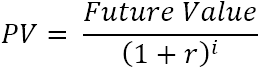Where:

• r – discount or interest rate
• i – the cash flow period

For example, to get \$110 (future value) after 1 year (i), how much should you invest today in your bank account which is offering 10% annual interest rate (r)? The above formula gives this answer:

`\$110/(1+10%)^1 = \$100`

In other words, \$100 is the present value of \$110 that are expected to be received in the future.

Net present value (NPV) adds up the present values of all future cashflows to bring them to a single point in present. And because the idea of "net" is to show how profitable the project is going to be after accounting for the initial capital investment required to fund it, the amount of initial investment is subtracted from the sum of all present values: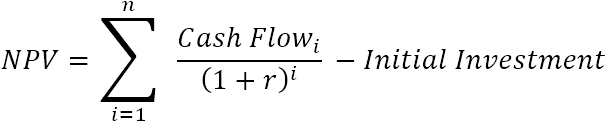Where:

• r – discount or interest rate
• n – the number of time periods
• i – the cash flow period

Because any non-zero number raised to the zero power equals 1, we can include the initial investment in the sum. Please notice, that in this compact version of the NPV formula, i=0, i.e. the initial investment is made in period 0.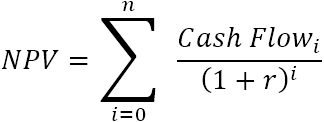For example, to find NPV for a series of cash flows (50, 60, 70) discounted at 10% and the initial cost of \$100, you can use this formula: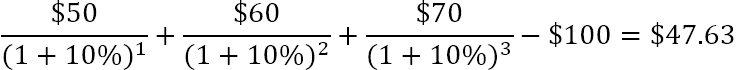Or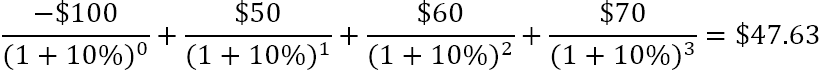How does the net present value help in evaluating a financial viability of a proposed investment? It is assumed that an investment with a positive NPV will be profitable, and an investment with a negative NPV will be unprofitable. This concept is the basis of the Net Present Value Rule, which says that you should only engage in projects with a positive net present value.

## Excel NPV function

The NPV function in Excel returns the net present value of an investment based on a discount or interest rate and a series of future cash flows.

The syntax of the Excel NPV function is as follows:

NPV(rate, value1, [value2], …)

Where:

• Rate (required) - the discount or interest rate over one period. It must be supplied as percentage or a corresponding decimal number.
• Value1, [value2], …- numeric values representing a series of regular cash flows. Value1 is required, subsequent values are optional. In the modern versions of Excel 2007 to 2019, up to 254 value arguments can be supplied; in Excel 2003 and older – up to 30 arguments.

The NPV function is available in Excel for Office 365, Excel 2019, Excel 2016, Excel 2013, Excel 2010, Excel 2007, Excel 2003, Excel XP, and Excel 2000.

### 4 things you should know about NPV function

To ensure that your NPV formula in Excel calculates correctly, please keep in mind these facts:

• Values must occur at the end of each period. If the first cash flow (initial investment) occurs at the beginning of the first period, use one of these NPV formulas.
• Values must be supplied in chronological order and equally spaced in time.
• Use negative values to represent outflows (cash paid out) and positive values to represent inflows (cash received).
• Only numerical values are processed. Empty cells, text representations of numbers, logical values, and error values are ignored.

### How Excel NPV function works

Using the NPV function in Excel is a bit tricky because of the way the function is implemented. By default, it is assumed that an investment is made one period before the value1 date. For this reason, an NPV formula in its pure form works right only if you supply the initial investment cost one period from now, not today!

To illustrate this, let's calculate net present value manually and with an Excel NPV formula, and compare the results.

Let's say, you have a discount rate in B1, a series of cash flows in B4:B9 and period numbers in A4:A9.

Supply the above references in this generic PV formula:

PV = future value/(1+rate)^period

And you will get the following equation:

`=B4/(1+\$B\$1)^A4`

This formula goes to C4 and is then copied to the below cells. Due to the clever use of absolute and relative cell references, the formula adjusts perfectly for each row as shown in the screenshot below.

Please notice that we calculate the present value of the initial investment too since the initial investment cost is after 1 year, so it is also discounted.

After that, we sum all the present values:

`=SUM(C4:C9)`

And now, let's do NPV with the Excel function:

`=NPV(B1, B4:B9)`

As you can see, the results of both calculations match exactly: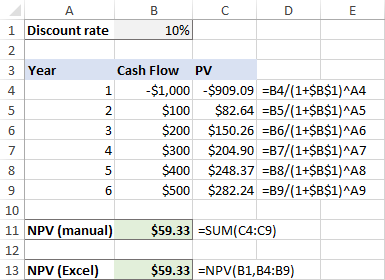But what if the initial outlay occurs at the start of the first period, as it typically does?

Because the initial investment is made today, no discounting applies to it, and we simply add this amount to the sum of the present values of future cash flows (since it's a negative number, it is actually subtracted):

`=SUM(C4:C9)+B4`

And in this case, the manual calculation and Excel NPV function yield different results: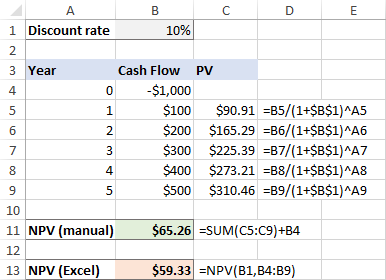Does this mean we cannot rely on the NPV formula in Excel and have to calculate net present value manually in this situation? Of course, not! You will just need to tweak the NPV function a little as explained in the next section.

## How to calculate NPV in Excel

When the initial investment is made at the start of the first period, we can treat it as a cash flow at the end of the previous period (i.e. period 0). With that in mind, there are two simple ways to find NPV in Excel.

### Excel NPV formula 1

Leave the initial cost out of the range of values and subtract it from the NPV function's result. Since the initial outlay is typically entered as a negative number, you actually perform the addition operation:

NPV(rate, values) + initial cost

In this case, the Excel NPV function just returns the present value of uneven cash flows. Because we want "net" (i.e. present value of future cash flows less initial investment), we subtract the initial cost outside of the NPV function.

### Excel NPV formula 2

Include the initial cost in the range of values and multiply the result by (1 + rate).

In this case, the Excel NPV function would give you the result as of period -1 (as if the initial investment was made one period before period 0), we have to multiply its output by (1 + r) to bring the NPV forward one period in time (i.e. from i = -1 to i = 0). Please see the compact form of the NPV formula.

NPV(rate, values) * (1+rate)

Which formula to use is a matter of your personal preference. I personally believe the first one is simpler and easier to understand.

### NPV calculator in Excel

Now let's see how you can use the above formulas on real data to make your own NPV calculator in Excel.

Supposing you have the initial outlay in B2, a series of future cash flows in B3:B7, and the required return rate in F1. To find NPV, use one of the following formulas:

NPV formula 1:

`=NPV(F1, B3:B7) + B2`

Please notice that the first value argument is the cash flow in period 1 (B3), the initial cost (B2) is not included.

NPV Formula 2:

`=NPV(F1, B2:B7) * (1+F1)`

This formula includes the initial cost (B2) in the range of values.

The below screenshot shows our Excel NPV calculator in action: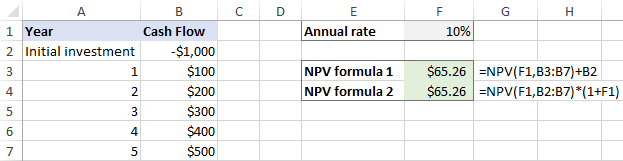To make sure our Excel NPV formulas are correct, let us check the result with manual calculations.

First, we find the present value of each cash flow by using the PV formula discussed above:

`=B3/(1+\$F\$1)^A3`

Next, add up all the present values and subtract the initial cost of investment:

`=SUM(C3:C7)+B2`

… and see that the results of all three formulas are absolutely the same.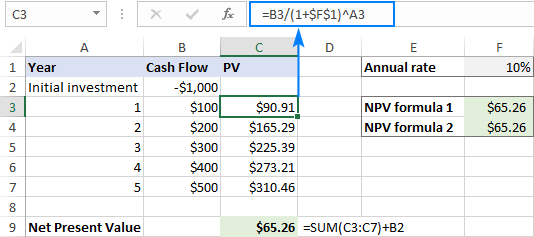Note. In this example, we are dealing with yearly cash flows and annual rate. If you are to find quarterly or monthly NPV in Excel, be sure to adjust the discounting rate accordingly as explained in this example.

## Difference between PV and NPV in Excel

In finance, both PV and NPV are used to discount future cash flows to the present to estimate the current value of future income. But they differ in one important way:

• Present value (PV) - only accounts for cash inflows.
• Net present value (NPV) – is the difference between the present value of cash inflows and the present value of cash outflows. It accounts for the initial outlay required to fund a project, making it a net figure.

In Microsoft Excel, there are two essential differences between the functions:

• The NPV function can calculate uneven (variable) cash flows. The PV function requires cash flows to be constant over the entire life of an investment.
• With NPV, cash flows must occur at the end of each period. PV can handle cash flows that occur at the end and at the beginning of a period.

## Difference between NPV and XNPV in Excel

XNPV is one more Excel financial function that calculates the net present value of an investment. The primary difference between the functions is as follows:

• NPV deems all time periods to be equal.
• XNPV allows you to specify dates that correspond to each cash flow. For this reason, the XNPV function is a lot more precise when dealing with a series of cash flows at irregular intervals.

Unlike NPV, the Excel XNPV function is implemented "normally" - the first value corresponds to the outflow that occurs at the beginning of the investment. All successive cash flows are discounted based on a 365-day year.

In terms of syntax, the XNPV function has one additional argument:

XNPV(rate, values, dates)

As an example, let's use both functions on the same data set, where F1 is the discount rate, B2:B7 are cash flows and C2:C7 are dates:

`=NPV(F1,B3:B7)+B2`

`=XNPV(F1,B2:B7,C2:C7)`

If the cash flows are distributed evenly through the investment, the NPV and XNPV functions return very close figures: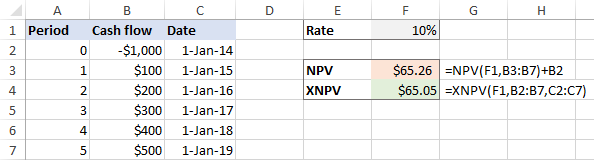In case of irregular intervals, the difference between the results is very significant: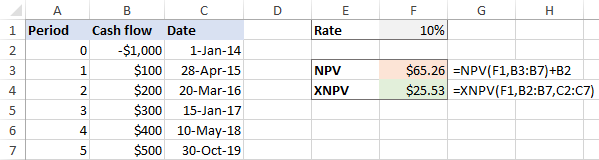## Common errors when calculating NPV in Excel

Because of a quite specific implementation of the NPV function, many errors are made when calculating net present value in Excel. The simple examples below demonstrate the most typical errors and how to avoid them.

### Irregular intervals

The Excel NPV function assumes that all cash flow periods are equal. If you supply different intervals, say years and quarters or months, the net present value will be incorrect because of non-coherent time periods.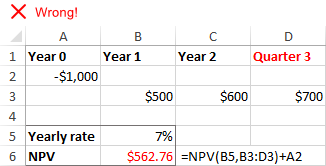### Missing periods or cashflows

NPV in Excel does not recognize omitted periods and ignores empty cells. To calculate NPV correctly, please be sure to provide consecutive months, quarters, or years and supply zero values for time periods that have null cash flows.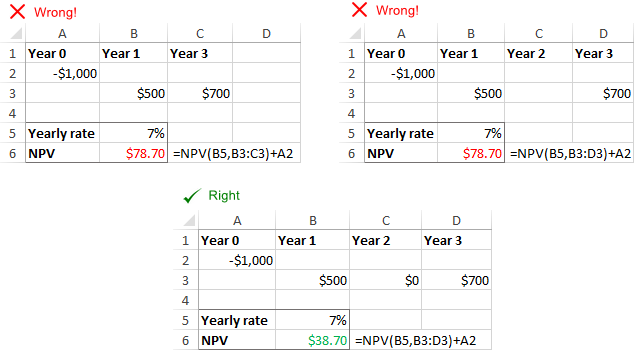### Discounting rate does not correspond to actual time periods

The Excel NPV function cannot adjust the supplied rate to the given time frequencies automatically, for example annual discounting rate to monthly cash flows. It is the user's responsibility to provide an appropriate rate per period.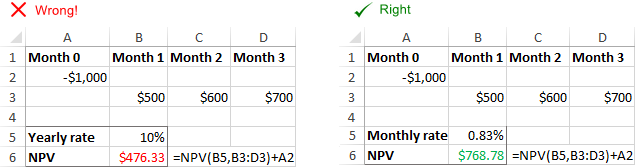### Incorrect rate format

The discount or interest rate must be provided as a percentage or corresponding decimal number. For example, the 10 percent rate can be supplied as 10% or 0.1. If you enter the rate as number 10, Excel will treat it as 1000%, and NPV will be calculated wrong.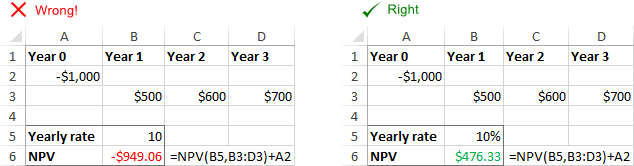That's how to use NPV in Excel to find the net present value of an investment. To have a closer look at the formulas discussed in this tutorial, please feel free to download our sample NPV calculator for Excel.

Thank you for reading and hope to see you on our blog next week!

### 2 responses to "How to calculate NPV in Excel - net present value formula examples"

1.Sal says:

From above comments. Under 'Discounting rate does not correspond to actual time periods'
It is incorrect to say that 'For this, you just need to divide the annual rate by the number of periods per year'
Correct way is, for example for quarterly payment, to adjust like this =(1+i)^(1/4)-1 (where i is the annual interest rate).

2.Darci says:

Wow, totally stuck on an inflow/outflow accounting questions for a couple of days now (it's not due yet), and all I had to do was make it a negative number 😲
THANKS!!